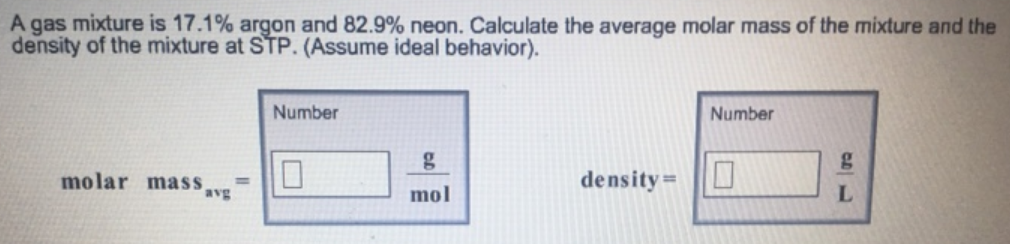# Problem: A gas mixture is 17.1% argon and 82.9% neon. Calculate the average molar mass of the mixture and the density of the mixture at STP. (Assume ideal behavior).

###### FREE Expert Solution
86% (420 ratings)###### Problem Details

A gas mixture is 17.1% argon and 82.9% neon. Calculate the average molar mass of the mixture and the density of the mixture at STP. (Assume ideal behavior).